# Novel Neural network single sensor MPPT for Proton Exchange Membrane Fuel Cell

Novel Neural network single sensor MPPT for Proton Exchange Membrane Fuel Cell
Mechatronics Laboratory, Optics and Precision Mechanics Institute, Ferhat Abbas University Setif 1, Cite Maabouda (ex. Travaux), 19000 Setif Algeria

Corresponding Author Email:
a.b.harrag@gmail.com
Page:
43-48
|
DOI:
https://doi.org/10.14447/jnmes.v24i1.a08
10 November 2020
|
Accepted:
31 January 2021
|
Published:
31 March 2021
| Citation
Abstract:

This paper presents a new neural network single sensor maximum power point tracking algorithm controlling the DC-DC boost converter to guarantee the transfer of the proton exchange membrane fuel cell maximum generated power to the load. The implemented neural network single sensor controller has been developed and trained firstly in offline mode using single sensor maximum power point tracking data obtained previously; and secondly used in online mode to track the maximum output power of the fuel cell power system. Comparative simulation results prove the superiority of the proposed neural network single sensor maximum power point compared to the single sensor one especially in transit response reducing by the way the overshoot and the tracking time which leads to an overall energy losses reduction. In addition, the implemented neural network single sensor MPPT employs only one sensor which will reduce the complexity and the cost of PEM fuel cell power system. To our knowledge, this study is a pioneering work using a neural network single sensor controller as PEM fuel cell MPPT.

Keywords:

PEM Fuel Cell, MPPT, Single Sensor, Neural Network, NN

1. Introduction

In the last decades, the demand for clean, green and sustainable energy sources has become a strong requirement and driving force in the continuity of economic development and therefore in the enhancement of human living conditions. Consequently, fuel cells and hydrogen energy in general have been acknowledged as one of keystones of clean energy technologies due to their high energy density, high efficiency, and low/zero emissions. Lately, diverse energy sectors like transportation, stationary and portable power, and micro-power have experiencing an explosive growth of applications using fuel cells requiring by the way a basic science and technology knowledge as well as advanced fuel cell design and analysis techniques .

A fuel cell is an electrochemical device converting continuously the chemical energy content of the fuel into electrical energy, water, and heat as long via reverse electrochemical reactions. Among various types of fuel cells, the high temperature solid oxide fuel cell (SOFC) and the low temperature proton exchange membrane fuel cell (PEMFC) have been identified as the expected fuel cell categories that will dominate the market in the near future. The PEMFC uses a solid membrane that transports protons. It can operate from about 0°C to 80°C with the output power ranging from a few watts to several hundred kilowatts.

One of most relevant issue in fuel cell usage is it's stability related to its non regulated output power due especially to the heavily influence of changes in electric current, temperature, membrane water content, stoichiometry, partial gas pressures, gas speed and reactants humidity level on its voltage. As a result, the fuel cell maximum power extraction is crucial for its economical and optimum usage. Conversely, due to the varying load current requirements and the varying operating conditions, the extraction of the maximum available power varies dynamically during the fuel cell operation making it as a challenging task.

The last decade has observed a vast implementation of fuel cell maximum power point tracking (MPPT) controllers [4-5], among them: perturb and observe, incremental conductance, sliding mode approach, fractional order filter strategy, hysteresis method, extremum seeking control, fuzzy logic controller[12-13], particle swarm optimization controller, water cycle algorithm, unified tracker algorithm , eagle strategy method , neural network approach[18-19], etc.

This paper presents a new neural network single sensor maximum power point tracking algorithm controlling the DC-DC boost converter to guarantee the transfer of the proton exchange membrane fuel cell maximum generated power to the load. The implemented neural network single sensor controller has been developed and trained firstly in offline mode using single sensor maximum power point tracking data obtained previously; and secondly used in online mode to track the maximum output power of the fuel cell power system. Comparative simulation results prove the superiority of the proposed neural network single sensor maximum power point compared to the single sensor one especially in transit response reducing by the way the overshoot and the tracking time which leads to an overall energy losses reduction. In addition, the implemented neural network single sensor MPPT employs only one sensor which will reduce the complexity and the cost of PEM fuel cell power system. The rest of this paper is structured as follows. the PEM fuel cell modelling is described in Section 2. Section 3 presents the neural network single sensor MPPT controller. Simulation results and discussions are presented in Section 4; while Section 5 concludes this study.

2. PEM Fuel Cell Modeling

A fuel cell is a simple electrochemical device that produces electricity along with water and heat using the chemical energy present in hydrogen and oxygen. More specifically, hydrogen is fed into the anode, where it is separated d into electrons and protons with the help of a catalyst. The electrons as they pass through the external circuit to reach the cathode provide the electrical current. The protons pass through the proton-conducting membrane and crossover into the cathode to recombine with the electrons as well as the oxygen (which is fed into the cathode) to generate water (Fig. 1) .

## 1.png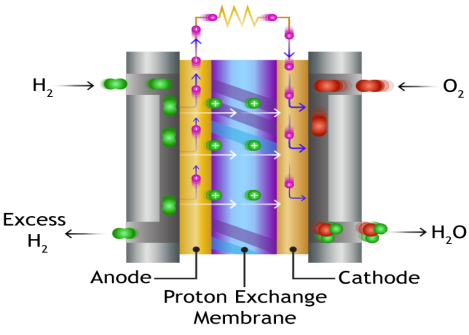Figure 1. PEM fuel cell.

Oxidation of hydrogen reaction at anode:

$\mathrm{H}_{2} \rightarrow 2 \mathrm{H}^{+}+2 \mathrm{e}^{-}$        (1)

Reduction of oxygen reaction at a cathode:

$\mathrm{O}_{2}+4 \mathrm{e}^{-} \rightarrow 2 \mathrm{O}^{-2}$       (2)

The overall hydrogen reaction is:

$\mathrm{H}_{2}+\frac{1}{2} \mathrm{O}_{2} \rightarrow \mathrm{H}_{2} \mathrm{O}+$ electricalenergy $+$ heat      (3)

Each cell voltage can be defined by the well known expression given by :

$\mathrm{V}_{\mathrm{FC}}=\mathrm{E}_{\text {nernst }}-\mathrm{V}_{\mathrm{act}}-\mathrm{V}_{\text {ohmic }}-\mathrm{V}_{\text {conc }}$       (4)

The reversible open circuit voltage Enernst is approximated by :

$\mathrm{E}_{\text {nernst }}=1.229-\left(8.5 \times 10^{-4}\right)(\mathrm{T}-298.15)+$

$\left(4.385 \times 10^{-5} \mathrm{~T}\left[\ln \left(\mathrm{P}_{\mathrm{H} 2}\right)+0.5 \ln \left(\mathrm{P}_{\mathrm{O} 2}\right)\right]\right.$        (5)

where T, PO2 and PH2 are the temperature, the oxygen pressure and the hydrogen pressure, respectively.

The activation voltage drop Vact is approximated by :

$V_{\text {act }}=\xi_{1}+\xi_{2} \cdot T+\xi_{3} \cdot T \cdot \ln \left(C_{02}\right)+\xi_{4} \cdot T \cdot \ln \left(i_{F C}\right)$     (6)

where xi(i = 1 to 4), iFC and CO2 are the parametric coefficients for each cell, the cell current and the oxygen’s concentration, respectively.

The ohmic linear voltage drop Vohmic is proportional to electric current approximated by :

$V_{\text {ohmic }}=\left(R_{c}+R_{m}\right) \cdot i_{F C}$       (7)

where iFC, Rc and Rm are the cell current, the contact resistance Rc and the membrane resistance, respectively.

The concentration voltage drop $\mathrm{V}_{\text {conc }}$ is approximated by :

$V_{\text {conc }}=-b \cdot \ln \left(1-\frac{i_{F C} / A}{I_{\max }}\right)$       (8)

where b, iFC, A and $\mathrm{I}_{\max }$ are the concentration loss constant, the cell current, the cell active area and the maximum current density, respectively.

3. Proposed Neural Network Single Sensor MPPT Algorithm

3.1 Methodology

The proposed neural network single sensor adjustable step MPPT is developed and trained, initially in offline mode required to fix the optimal neural network setting and architecture, used finally in online mode to track the PEM fuel cell power source maximum output power.

The developed controller uses the PEM fuel cell current as well as the old PWM ratio as inputs to compute the new PWM ratio, used as output. Figure 2 shows the proposed neural network architecture.

## 2.png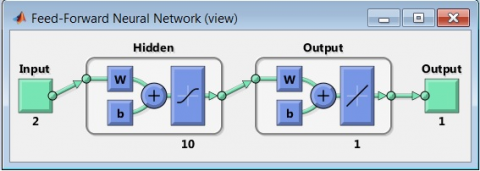Figure 2. Proposed neural network controller architecture.

3.2 Neural Network training

In this study, the mean squared errors (MSE) algorithm has been used to train the neural network controller by minimizing the overall error measure between the data generated previously using the conventional single sensor MPPT and the neural networks output. Figure 3 shows the MSE evolution.

3.3 Validation and Testing

Once trained, the optimized neural network controller has been used to track the maximum available power under different operating conditions considering temperature and hydrogen pressure changing. Figure 4 shows the validation process.

## 3.pngFigure 3. Mean squared errors

## 4.pngFigure 4. Neural network controller validation.

4. Results and Discussion

The Matlab/Simulink neural network single sensor maximum power point tracking algorithm implemented model controlling the DC-DC boost converter to ensure the transfer of the 7kW PEM fuel cell maximum generated power to a 50W resistive load is shown in Figure 5.

## 5.png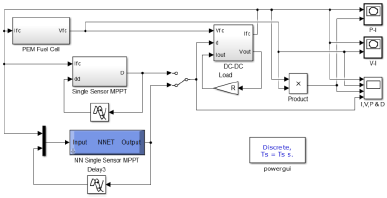The parameters of the DC-DC boost converter are given in Table 1; while the 7kW PEM fuel cell parameters are given in Table 2.

Table 1. DC-DC boost converter parameters

 Parameter Value C (µF) 1000 L (mH) 5 Resistive Load R (Ω) 50 Switching frequency Fs (kHz) 10

Table 2. 7kW PEM fuel cell parameters

 Parameter Value Maximum Power at MPP PMPP (W) 7000 Cell open circuit voltage VOC (V) 1.229 Cell active surface A (cm2) 200 Number of cells N 50 Oxygen partial pressure PO2 (bar) 0.3 Hydrogen partial pressure PH2 (bar) 2.6 Nominal voltage Vg (V) 47

The performance of the implemented neural network single sensor MPPT Matlab/Simulink model has been compared to the performance of the single sensor MPPT studied previously considering two test cases:

• Case 1: Fast temperature (T) changing;
• Case 2: Fast hydrogen pressure (PH2) changing.

The two considered test cases have been used to evaluate the efficiency and the capability tracking of the proposed MPPT controller by using a fast stepped pattern considered as strained testing cases.

4.1 Fast temperature (T) changing

In this case, we assess the tracking rapidity of the proposed neural network single sensor MPPT considering the fast temperature changing as shown in Figure 6.

## 6.png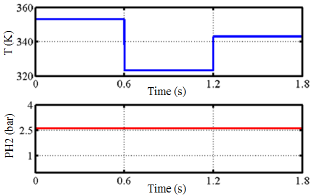Figure 6. Fast temperature changing profile.

Figure 7 shows the corresponding output power.

## 7.png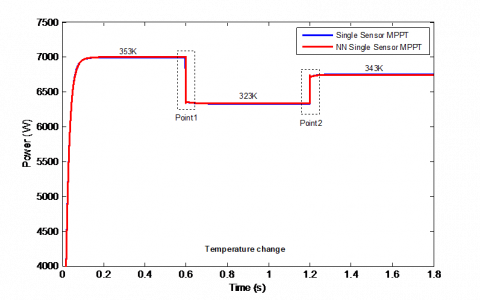Figure 7. Output power in case of fast temperature changing.

Figures 8 and 9 show the zoom-in of point 1 representing a decrease of the temperature from 353K to 323K at 0.6s and point 2 representing an increase of the temperature from 323K to 343K at 1.2s; while Fig. 10 gives the corresponding P-I characteristics.

## 8.png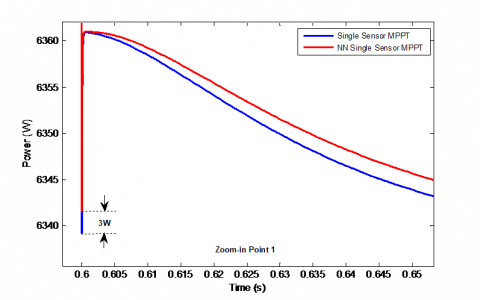Figure 8. Overshoot in case of fast temperature decrease from 353K to 323K (Point 1).

## 9.png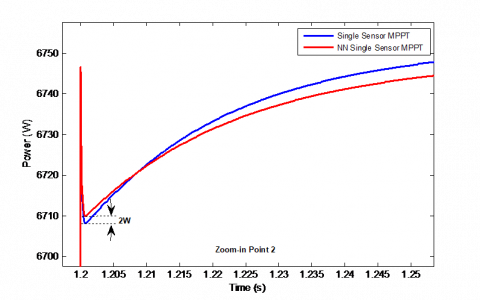Figure 9. Overshoot in case of fast temperature increase from 323K to 343K (Point 2).

## 10.pngFigure 10. P-I curves in case of fast temperature changing.

From Figures 8 and 9, the proposed neural network single sensor performs better compared to the single sensor one especially in case of fast temperature changing providing an overshoot reduction (3W for the point 1 and 2W for the point2). In addition, the proposed MPPT track effectively the maximum output power providing the shortest path reducing by the way the tracking time as well as oscillation around the maximum power point as shown in Figure 10.

4.2 Fast hydrogen pressure (PH2) changing

This case serve to evaluate the tracking efficiency of the proposed neural network single sensor MPPT in case of fast hydrogen pressure changing as shown in Figure 11.

Figure 12 shows the corresponding output power. While Figures 13 and 14 show the zoom-in of point A representing a decrease of the hydrogen pressure from 2.6bar to 2.1bar at 0.6s and point B representing an increase of the hydrogen pressure from 2.1bar to 2.6bar at 1.2s; while Figure 15 shows the corresponding P-I characteristics according to the considered pressure variation.

## 11.png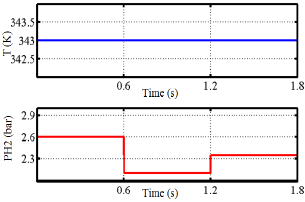Figure 11. Fast hydrogen pressure changing profile.

From Figures 12 and 13, the neural network single sensor provides an overshoot reduction around 3W for the points A and B representing a fast hydrogen pressure changing. Moreover, as shown in Fig. 14, the neural network single sensor MPPT tracks faster the available maximum power point by traversing the shortest path which reduces the convergence time and consequently reduces power losses.

From Figures 7 to 10 and 12 to 15, we can conclude that the performance of proposed neural network single sensor MPPT are superior to those of the single sensor MPPT regarding fast changing of temperature or hydrogen pressure leading to an overall energy losses reduction.

## 12.png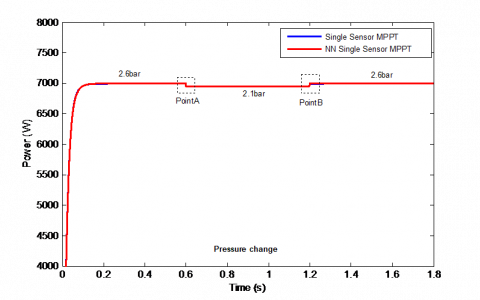Figure 12. Out power in case of fast hydrogen pressure changing.

## 13.png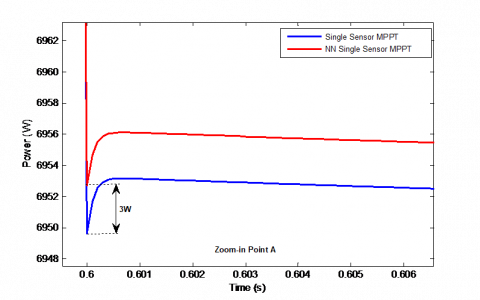Figure 13. Overshoot in case of fast hydrogen pressure decrease from 2.6bar to 2.1bar (Point A).

## 14.png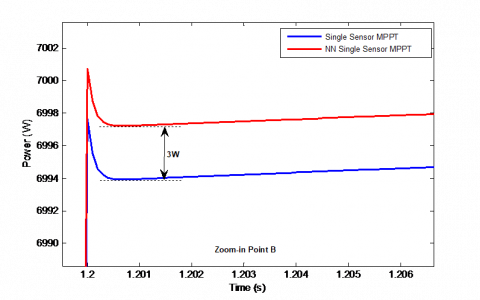Figure 14. Overshoot in case of fast hydrogen pressure increase from 2.1bar to 2.6bar (Point B).

## 15.pngFigure 15. P-I curves in case of fast hydrogen pressure changing.

5. Conclusions

This paper addresses the implementation of a novel neural network single sensor MPPT controlling the DC-DC boost converter to guarantee the transfer of a 7kW PEM fuel cell maximum generated power to a 50W resistive load. Comparative simulation results obtained using Matlab-Simulink software prove the superiority of the neural network single sensor maximum power point compared to the single sensor one especially in transit response reducing by the way the overshoot and the tracking time which leads to an overall energy losses reduction.In addition, the implemented neural network single sensor MPPT employs only one sensor which will reduce the complexity and the cost of PEM fuel cell power system. As future work, we work currently on the experimental validation of the developed neural network single sensor MPPT in the hardware in the loop mode using the STM32F4 board.

6. Acknowledgment

The Algerian Ministry of Higher Education and Scientific Research via the DGRSDT supported this research (PRFU Project code: A01L07UN190120180005).

Nomenclature
 V Voltage, V i Current, A T Temperature, K P Pressure, bar R Resistance, W A Active area, cm2 Greek symbols xi(i = 1 to 4) Parametric coefficients Subscripts FC Fuel Cell Nernst Nernst voltage act Activation losses ohmic Ohmic losses conc Concentration losses H2 Hydrogen O2 Oxygen C Contact M Membrane
References

 S. Revankar and P. Majumdar, Fuel cells: Principles, Design and Analysis, CRC Press, Taylor & Francis, Boca Raton, 2014.

 A. Qi, Proton Exchange Membrane Fuel Cells, CRC Press, Taylor & Francis, Boca Raton 2014.

 C.A. Ramos-Paja, G. Spagnuolo, G. Petrone, R. Giral and A. Romero, Fuel cell MPPT for fuel consumption optimization. In Proceedings IEEE International Symposium on Circuits and Systems (ISCAS), 2010, pp. 2199-2202.

 M. Becherif and D. Hissel, Int J Hydrogen Energy, 35(22), 1252 (2010).

 N. Karami, R. Outbib and N. Moubayed, Fuel Flow Control of a PEM Fuel Cell with MPPT. In Proceedings IEEE International Symposium on Intelligent Control (ISIC), 2012. pp. 289-94.

 M. Sarvi and M. M. Barati, Voltage and current based MPPT of fuel cells under variable temperature conditions, 45th International Universities Power Engineering Conference UPEC2010, Cardiff, Wales, 2010, pp. 1-4.

 M.Z. Romdlony, B.R. Trilaksono and R. Ortega, Experimental study of extremum seeking control for maximum power point tracking of PEM fuel cell. In: International Conference on System Engineering and Technology (ICSET), 2012, pp. 1-6.

 S.H. Abdi, K. Afshar, N. Bigdeli, S. Ahmadi, Int J Electrochem Sci, 7, 4192 (2012).

 J. Liu, T. Zhao and Y. Chen, Maximum power point tracking of proton exchange membrane fuel cell with fractional order filter and extremum seeking control. In: Proceedings ASME/IEEE International Conference on Mechatronic and Embedded Systems and Applications (ICMESA), 2015, pp. 1-6.

 J.D. Park and Z. Ren, J Power Sources, 205, 151 (2012).

 K. Ettihir, L. Boulon, K. Agbossou and S. Kelouwani,  MPPT Control strategy on PEM fuel cell low speed vehicle. In: Proceedings IEEE Vehicle Power and Propulsion Conference (VPPC); 2012, pp. 926-931.

 J. Jiao and X.A. Cui, J Comput Inf Syst, 9(5), 1933 (2013).

 A. Harrag and S. Messalti, International Journal on Hydrogen Energy, 43(1), 537 (2018).

 I. Soltani, M. Sarvi and H. Marefatjou, World Appl Program, 3(7), 264 (2013).

 I.N. Avanaki and M. Sarvi, J Renew Energy Environ, 3(1) 35 (2016).

 H. Fathabadi, Energy, 116, 402 (2016).

 M. Sarvi, M. Parpaei, I. Soltani and M.A. Taghikhani, IJE Trans A Basics, 28(4), 529 (2015).

 A. Harrag and H. Bahri, Int. Journal of Hydrogen Energy, 42(5), 3549 (2017).

 M. Hatti, M. Tioursi and W. Nouibat, Neural network approach for semi-empirical modeling of PEM fuel-cell, In. IEEE International Symposium on Industrial Electronics 2006, pp. 1858-1863.

 C. Spiegel, PEM modeling and simulation using matlab. Burlington, MA: Academic Press, 2008.

 R. O'Hayre, Fuel Cell Fundamentals, John Wiley & Sons Ltd, 2009.

 R.F. Mann, J.C. Amphlett, M.A. Hooper, H.M. Jensen, B.A. Peppley and P.R. Roberge, Journal of Power Sources, 86(1-2), 173 (2000).

 D. Yu and S. Yuvarajan, A novel circuit model for PEM fuel cells, IEEE 19th Applied Power Electronics Conference and Exposition, , Anaheim, CA, USA, 2004, pp. 362-366.

 J.H. Lee, T.R. Lalk and A.J. Appleby, Journal of Power Sources, 73(2), 229 (1998).

 H.J. Avelar, E.A.A. Coelho, J.R. Camacho, J.B. Vieira, L.C. Freitas and M. Wu, PEM fuel cell dynamic model for electronic circuit simulator, IEEE Electrical Power & Energy Conference, Montreal, QC, Canada, 2009, pp. 1-6.

 A. Harrag and H. Bahri, Fuel Cells, 19(2), 177 (2019).